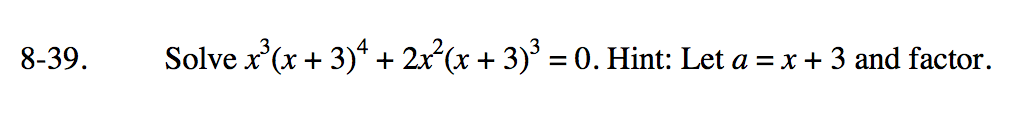### Home > PC3 > Chapter 8 > Lesson 8.1.2 > Problem8-39

8-39.

Solve x3(x + 3)4 + 2x2(x + 3)3 = 0. Hint: Let a = x + 3 and factor. Homework Help ✎Substitute a for x + 3.

Factor.

Set each factor equal to 0.

Substitute (x + 3) in each equation and solve.

x3a4 + 2x2a3 = 0

x2a3(ax + 2) = 0

x2a3 = 0 or ax + 2 = 0

x2(x + 3)3 = 0
x2 = 0
x = 0
(x + 3)3 = 0
x = −3

ax + 2 = 0
(x + 3)x + 2 = 0
x2 + 3x + 2 = 0
(x + 2)(x + 1) = 0
x = −2; x = −1